2018-05-06 15:12:33 qithon 阅读数 6756
• ###### Java经典算法讲解

在面试中，算法题目是必须的，通过算法能够看出一个程序员的编程思维，考察对复杂问题的设计与分析能力，对问题的严谨性都能够体现出来。一个算法的好坏，直接影响一个方法调用的性能，进而影响软件的整体性能。算法是学习所有编程语言的基础，在Java的学习过程中首先也会选择以算法起步，本次课程重点讲解Java开发中常用的基本算法。

30466 人正在学习 去看看 张中强

Definition : 约束多目标优化问题

$\begin{matrix} F(x)=(f_1(x),...,f_m(x))\;\;\;\;\;\;\;\;\;\;\\ s.t.\qquad\qquad\qquad\qquad\qquad\qquad \\ x_i^{(L)}\leq x_i \leq x_i^{(U)},\; i=1,2,...,n\;\\ g_j(x)\leq 0,\;j=1,2,...,J\;\;\;\;\;\;\;\;\;\;\;\\ h_k(x)=0,\;k=1,2,...,K\;\;\;\;\;\;\;\;\;\;\\ \end{matrix}$

$CV(x)=\sum_{i=1}^J{\langle g_j(x) \rangle} + \sum_{k=1}^K{|h_k(x)|}$

1. $x$是可行解，而$y$是不可行解；
2. $x,y$都不是可行解，但$CV(x)
3. $x,y$都是可行解，且$x$ Pareto支配 $y$

1. $x$是不可行解，$y$是可行解；
2. $x,y$都不是可行解，但$CV(x)>CV(y)$
3. $x,y$都是可行解，但解$y$的聚合函数值更小

QQ交流群：399652146

1. Deb K, Pratap A, Meyarivan T. Constrained Test Problems for Multi-objective Evolutionary Optimization[C]// Evolutionary Multi-Criterion Optimization, First International Conference, EMO 2001, Zurich, Switzerland, March 7-9, 2001, Proceedings. 2001:284–298.
2. Jain H, Deb K. An Evolutionary Many-Objective Optimization Algorithm Using Reference-Point Based Nondominated Sorting Approach, Part II: Handling Constraints and Extending to an Adaptive Approach[J]. IEEE Transactions on Evolutionary Computation, 2014, 18(4):602-622.
3. Li K, Deb K, Zhang Q, et al. An Evolutionary Many-Objective Optimization Algorithm Based on Dominance and Decomposition[J]. IEEE Transactions on Evolutionary Computation, 2015, 19(5):694-716.

2018-10-05 12:35:25 qithon 阅读数 1277
• ###### Java经典算法讲解

在面试中，算法题目是必须的，通过算法能够看出一个程序员的编程思维，考察对复杂问题的设计与分析能力，对问题的严谨性都能够体现出来。一个算法的好坏，直接影响一个方法调用的性能，进而影响软件的整体性能。算法是学习所有编程语言的基础，在Java的学习过程中首先也会选择以算法起步，本次课程重点讲解Java开发中常用的基本算法。

30466 人正在学习 去看看 张中强

MOEAPlat简介
github地址：https://github.com/qshzhang/MOEAs

QQ交流群：399652146

2017-06-06 16:54:59 qithon 阅读数 38140
• ###### Java经典算法讲解

在面试中，算法题目是必须的，通过算法能够看出一个程序员的编程思维，考察对复杂问题的设计与分析能力，对问题的严谨性都能够体现出来。一个算法的好坏，直接影响一个方法调用的性能，进而影响软件的整体性能。算法是学习所有编程语言的基础，在Java的学习过程中首先也会选择以算法起步，本次课程重点讲解Java开发中常用的基本算法。

30466 人正在学习 去看看 张中强

Definition 1: 多目标优化问题(multi-objective optimization problem(MOP))
$F(x)=(f_1(x),...,f_m(x))\\ s.t. \; x\in \Omega$

Definition 2: Pareto支配(Pareto Dominance)
x支配y,记为 x $\prec$ y ，当且仅当$\forall {i} \in \{1,2,...,m\}$,$f_i(x) \leq f_i$(y), 且$\exists {j} \in \{1,2,...,m\}$, s.t. $\; f_j(x)

Definition 3: Pareto最优解(Pareto Optimal Solution)

Definition 4: Pareto 集(Pareto Set)

Definition 5: Pareto 前沿(Pareto Front)
Pareto Set 中每个解对应的目标值向量组成的集合称之为Pareto Front, 简称为PF

Definition 6：近似集(Approximation Set)

Definition 7： 近似前沿(Approximation Front)
Approximation Set 中每个解对应的目标值向量组成的集合称之为Approximation Front 。

Definition 7：收敛性(Convergence)
Approximation Front 与 PF 的贴近程度。

Definition 8: 分布性(Distribution)

• 基于Pareto支配关系
• 基于分解的方法
• 基于Indicator方法

NSGA-II)，该方法主要包括快速非支配排序，将每次交叉突变产生的解和前一代的解合并，然后利用非支配排序分层，其伪代码如下：

FOR each $p\in P$
$\quad$ $S_p=\varnothing$
$\quad$ $n_p=0$
$\quad$ FOR each $q\in P$
$\qquad$ IF $p\prec q$
$\quad$$\qquad$ $S_p=S_p\bigcup \{q\}$
$\qquad$ ELSEIF $q\prec p$
$\quad$ $\qquad$ $n_p=n_p+1$
$\qquad$ ENDIF
$\quad$ ENDFOR
$\quad$ IF $n_p==0$
$\qquad$ $p_{rank}=1$
$\qquad$ $F_1=F_1\bigcup \{p\}$
$\quad$ ENDIF
ENDFOR
$i=1$
WHILE $F_i\neq \varnothing$
$\quad$ $Q=\varnothing$
$\quad$ FOR each $p\in F_i$
$\qquad$ FOR each $q\in S_p$
$\quad$ $\qquad$ $n_q=n_q-1$
$\quad$ $\qquad$ IF $nq==0$
$\qquad$ $\qquad$ $q_{rank}=i+1$
$\qquad$ $\qquad$ $Q=Q\bigcup \{q\}$
$\quad$ $\qquad$ ENDIF
$\qquad$ ENDFOR
$\quad$ ENDFOR
$\quad$ $i=i+1$
$\quad$ $F_i=Q$
ENDWHILE

$r=|Rt|$
for each $j$,set $Rt[j]_{distance}=0$
FOR each objective $m$
$\quad$ $Rt=sort(Rt,m)$
$\quad$ $Rt_{distance}=Rt[r]_{distance}=\infty$
$\quad$ FOR $j=2:(r-1)$
$\qquad$ $Rt[j]_{distance}=Rt[j]_{distance}+(Rt[j+1].m-Rt[j-1].m)/(f_m^{max}-f_m^{min})$
$\quad$ ENDFOR
ENDFOR

• Weighted Sum Approach
• Tchebycheff Approach
• penalty-based boundary intersection (PBI) approach

Weighted Sum Approach
$min \; g^{ws}(x|\lambda)=\sum_{i=1}^m\lambda_if_i(x)$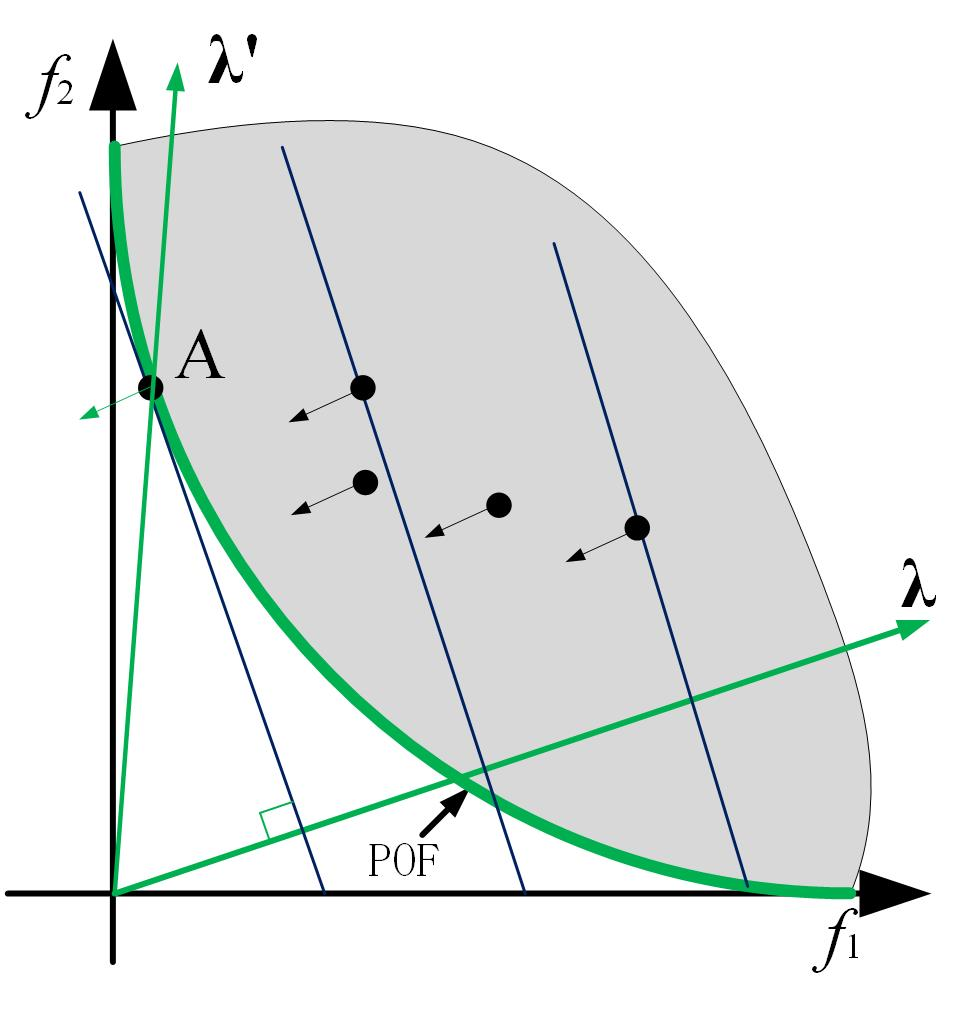Tchebycheff Approach
$min\; g^{te}(x|\lambda,z^*)=max\; \{\lambda_i (f_i(x) - z_i^*)\}$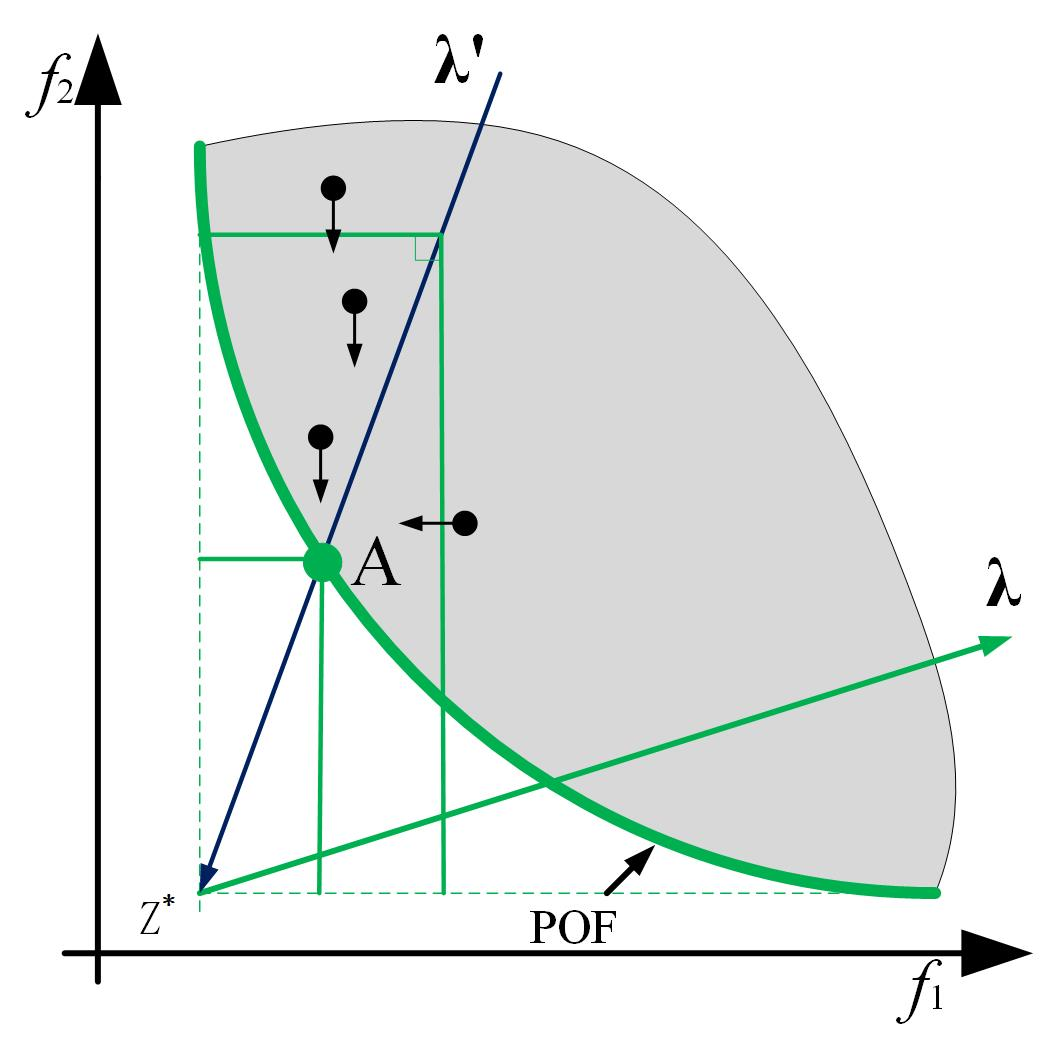penalty-based boundary intersection (PBI) approach
$min\; g^{pbi}(x|\lambda,z^*)=d_1+\theta d_2$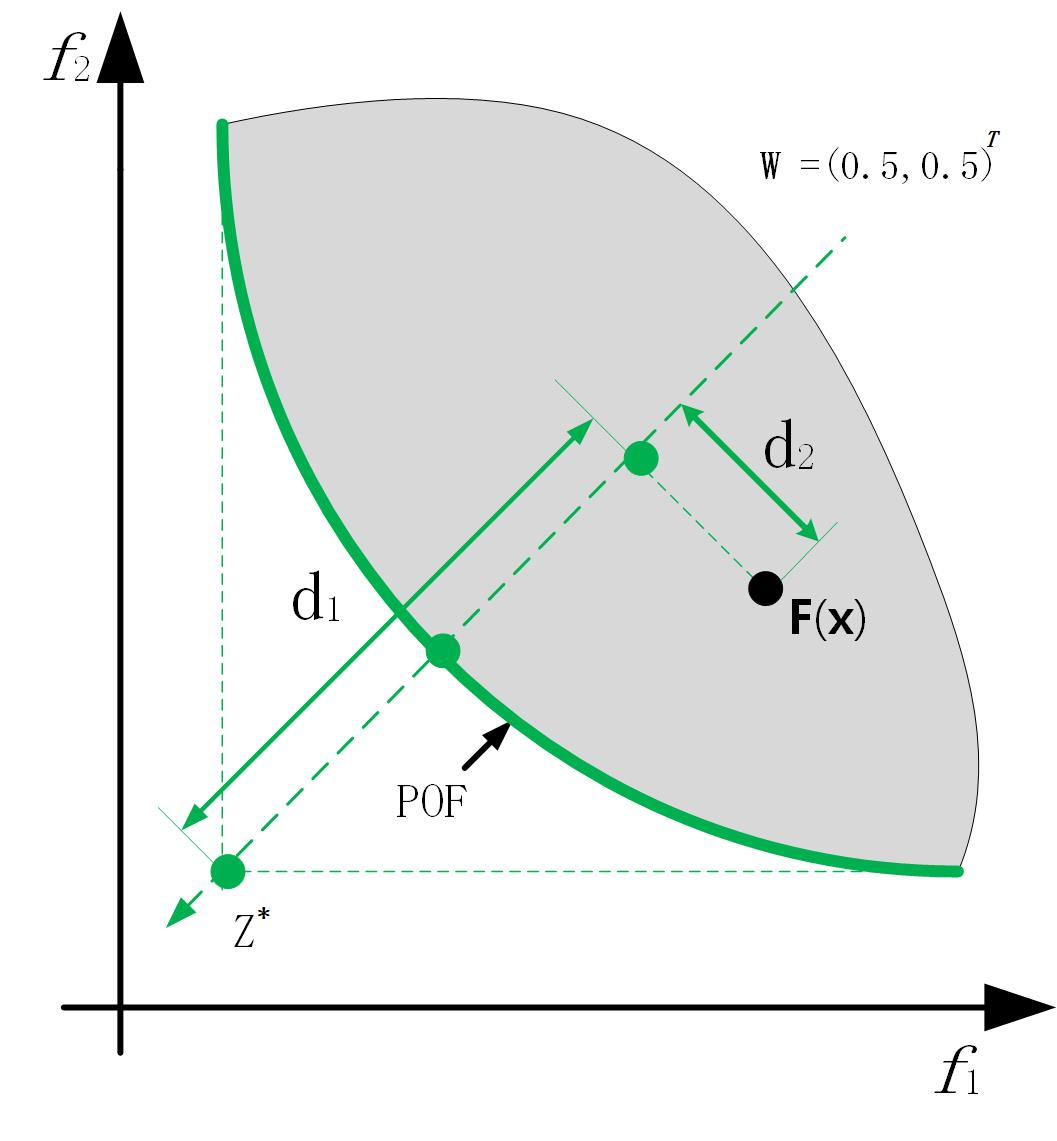MOEA/D算法流程

$N$个均匀分布的权重向量：$\lambda_1,\lambda_2,...,\lambda_N$ // $N$ 是种群大小
$P_0$：随机初始化的种群
$z^*$：初始化的理想点
$T$：每个权重向量的邻居的个数
$B(i)=(i_1,i_2,...,i_T)$是第$i$个权重向量的$T$个邻居的编号
$t = 0$
WHILE $t < MaxIteration$
$\quad$ FOR($i=1,2,...,N$)
$\qquad$构造新解：$B(i)$中随机选择两个索引$k,l$，再基于$x^k,x^l$生成新解$y$
$\qquad$更新$z^*$对于$j=1,2,...,m$,如果$z_j^*，则用$f_j(y)$取代$z_j^*$
$\qquad$更新近邻解：对于每一个$j\in B(i)$，如果$g(y|\lambda_j,z^*)\leq g(x^j|\lambda_j,z^*)$，那么则用解$y$取代$x^j$
$\quad$ ENDFOR
$\quad$$t = t + 1$
ENDWHILE

ps：文献是我的硕士论文，里面有较为详细的综述类的描述，对多个经典算法以及一些遗传操作都有介绍，还有衡量指标也是。

QQ交流群：399652146

 K. Deb, A. Pratap, S. Agarwal, and T. Meyarivan, “A fast and elitist multiobjective genetic algorithm: NSGA-II,” IEEE Trans. Evol. Comput., vol. 6, no. 2, pp. 182–197, Apr. 2002
 E. Zitzler, M. Laumanns, and L. Thiele, “SPEA2: Improving the strength Pareto evolutionary algorithm,” in Proc. Evol. Methods Design Optim. Control Appl. Ind. Prob., Athens, Greece, 2002, pp. 95–100.
 Qingfu Zhang and Hui Li. Moea/d: A multiobjective evolutionary algorithm based on decomposition. IEEE Transactions on evolutionary computation, 11(6):712–731, 2007.
 Yutao Qi, Xiaoliang Ma, Fang Liu, Licheng Jiao, Jianyong Sun, and Jianshe Wu. MOEA/D with adaptive weight adjustment. Evolutionary computation, 22(2):231–264, 2014.
 Kalyanmoy Deb and Himanshu Jain. An evolutionary many objective optimization algorithm using reference- point-based nondominated sorting approach, part i: Solving problems with box constraints. IEEE Trans. Evolutionary Computation, 18(4):577–601, 2014.
 Yuan Yuan, Hua Xu, Bo Wang, and Xin Yao. A new dominance relation-based evolutionary algorithm for many-objective optimization. IEEE Transactions on Evolutionary Computation, 20(1):16–37, 2016.
 Indraneel Das and John E Dennis. Normal-boundary intersection: A new method for generating the pareto surface in nonlinear multicriteria optimization problems. SIAM Journal on Optimization, 8(3):631–657, 1998
 E. Zitzler and S. Kunzli, "Indicator-based selection in multiob- jective search,"in Proceedings of the 8th International Conference on Parallel Problem Solving from Nature, 2004, pp. 832–842.
 J. Bader and E. Zitzler, “HypE: An algorithm for fast hypervolume-based many-objective optimization,” Evolutionary Computation, vol. 19, no. 1, pp. 45–76, 2011.
 Tian Y, Cheng R, Zhang X, et al. An Indicator Based Multi-Objective Evolutionary Algorithm with Reference Point Adaptation for Better Versatility[J]. IEEE Transactions on Evolutionary Computation, 2017, PP(99):1-1.
 张作峰. 基于分解的多目标进化算法研究[D]. 湘潭大学, 2013.
 基于分解思想的多目标进化算法研究. 湖南大学, 2018

2016-09-10 11:29:02 ouyang______ 阅读数 227
• ###### Java经典算法讲解

在面试中，算法题目是必须的，通过算法能够看出一个程序员的编程思维，考察对复杂问题的设计与分析能力，对问题的严谨性都能够体现出来。一个算法的好坏，直接影响一个方法调用的性能，进而影响软件的整体性能。算法是学习所有编程语言的基础，在Java的学习过程中首先也会选择以算法起步，本次课程重点讲解Java开发中常用的基本算法。

30466 人正在学习 去看看 张中强

基本概念

EA(evolutionary algorithm) 进化算法

MOEA（多目标进化算法）（multi-objective evolutionary algorithm）

MOGA（多目标遗产算法）（multi-objective genetic algorithm）

EMOO（进化多目标优化）（evolutionary multi-objective optimization）

MOEA包括：NSGA-||、NPGA、SPEA2等

单目标选法

Pareto最优边界：

2018-03-24 14:57:31 qithon 阅读数 8330
• ###### Java经典算法讲解

在面试中，算法题目是必须的，通过算法能够看出一个程序员的编程思维，考察对复杂问题的设计与分析能力，对问题的严谨性都能够体现出来。一个算法的好坏，直接影响一个方法调用的性能，进而影响软件的整体性能。算法是学习所有编程语言的基础，在Java的学习过程中首先也会选择以算法起步，本次课程重点讲解Java开发中常用的基本算法。

30466 人正在学习 去看看 张中强

NSGA-II由Kalyanmoy Deb等人于2002年在文章"A Fast and Elitist Multiobjective Genetic Algorithm: NSGA-II"中提出，是对1994年提出的NSGA的改进，相比于NSGA，NSGA2的改进主要两点：

1. 提出一种快速非支配排序，使得Pareto支配排序的时间复杂度由$O(N^3)$优化到$O(N^2)$
2. 提出一种拥挤距离来衡量解的分布性，并基于此选择种群中的合适的个体

NSGA-II的提出，为多目标进化算法的一大进步，特别是其基于Pareto支配关系的框架被后来许多算法采用，如NSGA-III，VaEA等。同时，NSGA-II对于低维多目标优化问题效果是不错的，但是对于高维多目标优化问题，其首先面对的便是由于其基于Pareto支配关系所导致的选择压力过小的问题，其次，便是拥挤距离在高维空间不适用，计算复杂度也比较高。

MOEA/D由张青富老师等人于2007年在文章"MOEA/D: A Multiobjective Evolutionary Algorithm Based on Decomposition"中提出。MOEA/D将一个多目标优化问题转换为多个标量子问题，且每一个子问题由一个均匀分布的权重向量构成，对于每生成一个新解，则基于聚合函数对该子问题附近的解进行替换。MOEA/D的优时如下：

1. 收敛更快，计算复杂度低；
2. 对于比较简单的PF，由于指导进化的权重向量是均匀分布的，因此MOEA/D所得到的解分布比较均匀

MOEA/D的提出为多目标进化算法提供了一种新的解决方案，基于MOEA/D的算法随后遍地开花，MOEA/DD，MOEA/D-STM，MOEA/D-SAS等。标准的MOEA/D对于低维简单PF的多目标优化问题效果是很好的，但对于复杂PF的问题，其分布性将会大打折扣，同时，对于高维多目标优化问题，其分布性也不能得到保证，效果也比较差。

1. 从低维多目标优化问题(2-,3-目标)看：
低维情况也要分两种情况考虑，一种是简单的PF的MOP，一种是复杂PF的MOP。
首先来看简单的PF的情况。以2维的DTLZ1为例，下面两张图分别是NSGA-II和MOEA/D得到的2维DTLZ1的散点图。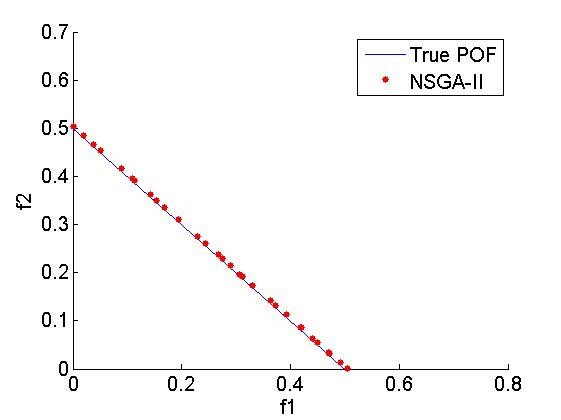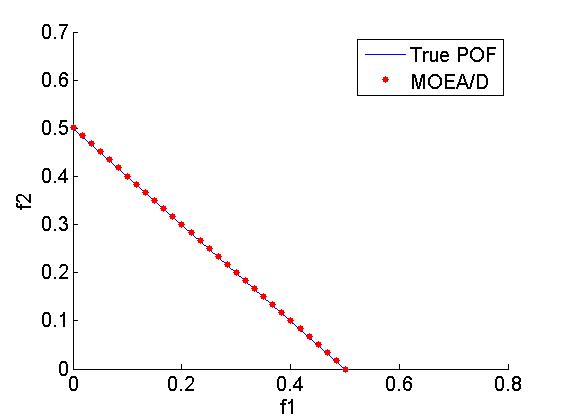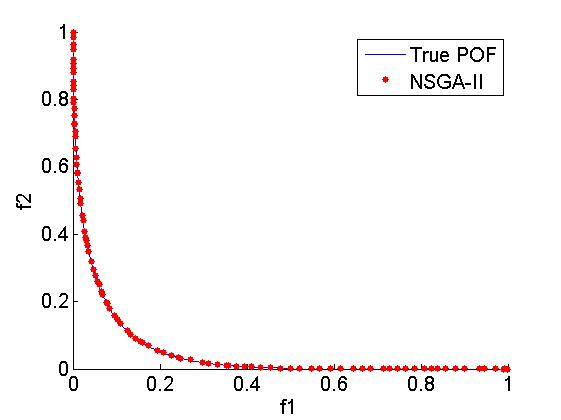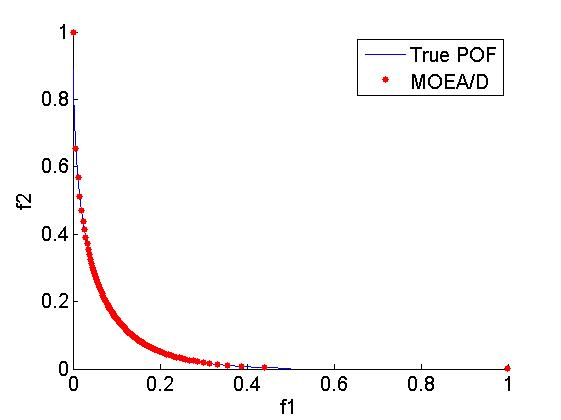3. 从高维看
NSGA-II 首先存在选择压力过小的问题 再就是拥挤距离的计算及其复杂
MOEA/D 在高维情况下分布性也不是很好

QQ交流群：399652146